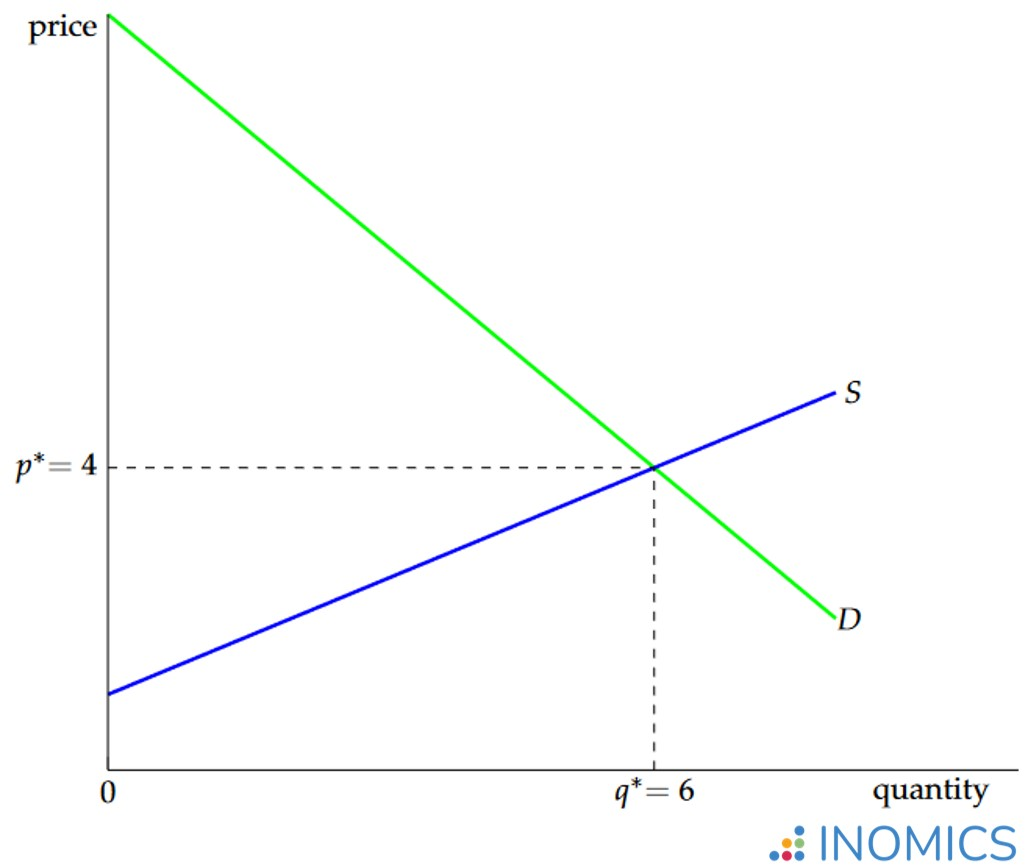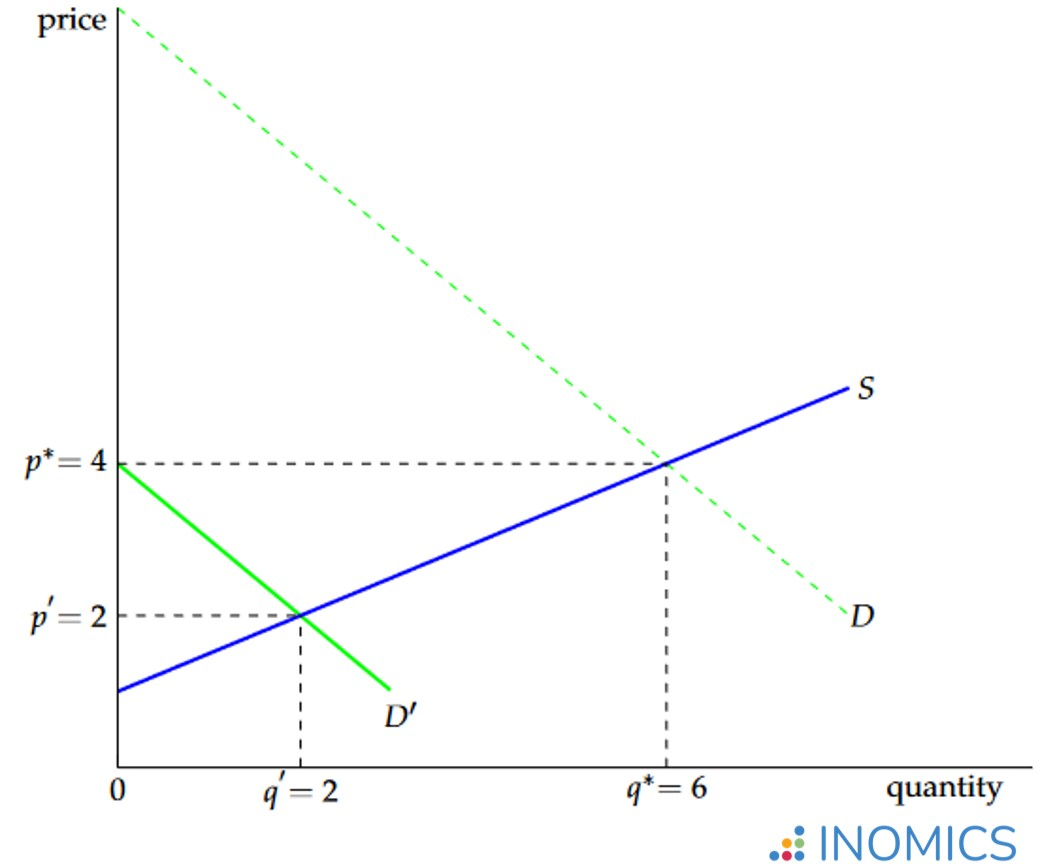# Market Equilibrium

Read a summary or generate practice questions based on this article with the new INOMICS AI toolhere.

By , reviewed by Sahar Milani

A market is in equilibrium if at the market price the quantity demanded is equal to the quantity supplied. The price at which the quantity demanded is equal to the quantity supplied is called the equilibrium price or market clearing price, and the corresponding quantity is the equilibrium quantity.

In a market, sellers who offer a good or service interact with buyers who do not possess the good and want to acquire it. At each price the sellers decide how many units they want to offer or supply at this price, and the buyers decide how many units they want to buy or demand. The quantity supplied will be higher the higher the market price of the good, whereas the quantity demanded will be lower the higher the market price of the good.

At the price at which these two quantities are identical, i.e., at the price at which the quantity demanded equals the quantity supplied, the market is in equilibrium. In equilibrium there are no buyers who would like to buy the good but cannot find a seller, and there are no sellers who would like to sell the good but are unable to find a buyer. This means that at the equilibrium price, the sellers are able to sell exactly the quantity they want to sell at this price and the buyers are able to buy exactly the quantity that they want to buy at this price.

If we know the demand and supply in a particular market, we can easily find the market equilibrium by looking for the price at which the quantity demanded is equal to the quantity supplied. For example, suppose that in the market for pencils the market demand is given by the linear demand function q= 10 - p and the market supply is equal to  q= 22. In equilibrium the number of pencils that the sellers want to sell has to be equal to the number of pencils that the buyers want to buy, i.e., quantity supplied has to be equal to the quantity demanded, or qqS. For the demand and supply function of our example this means

10 - p = 2p 2.

Now we only have to solve p to find the equilibrium price which is equal to p= 4. To find the corresponding quantity, we plug the equilibrium price p* back into the supply or the demand function and obtain q= 6. Thus, in our market for pencils the equilibrium price is equal to 4, and at this price the quantity exchanged is equal to 6 units.

This result is also shown in the graph below. The market equilibrium in a perfectly competitive market corresponds to the point of intersection of the supply curve and the demand curve. On the x-axis we have the quantity q of the good or service (in our case pencils) and on the y-axis the price p of the good. The green line represents the demand curve and shows the quantity demanded at each price (for the graph below the demand function is the same as in the example q= 10 - p). The blue line represents the supply curve (also taken from the example above qS = 2p 2) and shows the quantity supplied at each price. At the equilibrium price p= 4, the quantity demanded is equal to the quantity supplied (qD qq= 6).### Figure 1: Competitive market equilibrium

The market equilibrium is also called the competitive equilibrium, because it describes the allocation of goods and services in a perfectly competitive market (see the term for Perfect Competition). In a competitive market where buyers and sellers are price takers, the equilibrium price (and thus marginal revenue) will be equal to marginal costs and each firm makes a profit of zero.

The intuition behind this result is that in a perfectly competitive market without barriers to entry, firms will enter as long as they can make a positive profit. As the number of firms increases the market price decreases because new firms introduce excessive supply that cannot be sold at the original market price. Then they must lower prices to sell their stock. This decreases profits until they reach zero and firms have no incentive to enter the market anymore.## Excess demand and excess supply

If the market price is not equal to the equilibrium price, the quantity demanded is not equal to the quantity supplied. If the market price is too high (i.e. higher than the equilibrium price), many sellers want to sell, but only few buyers are interested in buying. This means that the quantity supplied exceeds the quantity demanded - a situation called excess supply. Coming back to our example: at the price = 6 per pencil, the quantity that sellers want to sell is equal to 2(6) - 2 = 10, while the quantity demanded is equal to 4 and there is an excess supply of 10 - 4 = 6 units.

So what will happen in this market? There are many sellers who would like to sell at the market price of 6, but are unable to find a buyer. Some of them will start lowering the price in order to be able to sell, because it is still better for them to sell pencils at a price of 5 euros (for example) than not sell anything at all. Sellers will continue to lower their price until the market price reaches 4, the equilibrium price. At this point sellers have no incentive to further reduce their price, because at the price of 4, they can sell exactly the number of units that they want to sell at this price.

Conversely, if the market price is too low, many buyers will be interested in acquiring the good, but few sellers will want to sell the good. This implies that the quantity demanded exceeds the quantity supplied and we call this situation excess demand. We can illustrate this situation using our example demand and supply equations. If the market price is equal to 2, the quantity demanded is equal to 8, but the quantity supplied is only equal to 2.

The price is so low that it is not profitable for the sellers to sell more than 2 units. This implies that some customers who want to buy the good cannot, because there is no seller who wants to sell to them at the market price. Consequently, the sellers, knowing that there is enough interest in their pencils, will increase their prices until the market price reaches the equilibrium price. In equilibrium, when the quantity demanded equals the quantity supplied, there is neither excess supply nor excess demand.

Whenever the market is not in equilibrium, either because the market price is too high (excess supply) or too low (excess demand), the forces of supply and demand will cause prices to adjust and the market price will move towards the equilibrium price.

In the description above we focused on the market equilibrium in a single market. We ignored that in reality there are many markets, one for each good or service that people want to trade. For this reason, what we described in the above article is also sometimes referred to as “partial equilibrium”, because it refers to the equilibrium for only one good and the rest of the economy might not be in equilibrium.

General equilibrium theory, on the other hand, takes the interaction between different markets into account and studies how the forces of supply and demand tend to move the economy as a whole towards an equilibrium. The equilibrium in general equilibrium theory is also sometimes called Walrasian equilibrium, named after the French mathematician and economist Léon Walras (1834-1910) who was the first to study and formalize this idea (see Walras, L., “Elements of pure Economics”).

## Good to know

Are the prices we observe when we enter a store competitive equilibrium prices? Not always. First of all, many markets are not perfectly competitive (i.e. some firms or consumers have market power and therefore the prices in these markets are not the prices we would expect to see in a perfectly competitive market).

Second, the equilibrium price of a specific good or service depends on many factors (e.g., the price of related goods, i.e. complements and substitutes, the income of the consumers, the costs of the producers, tastes, expectations, and so forth). Many of these factors affect the supply or demand function and change frequently over time. Therefore, the market prices of the goods and services also fluctuate over time and the prices we observe in the shops might be above or below the equilibrium price. But, the situations of excess supply or excess demand are only temporary, and as we know that the prices will move towards the equilibrium price, we expect that the prices we observe will fluctuate around the equilibrium price.

You need to login to comment